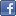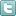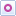EE Times-India > T&M

T&M# How to measure frequency response on a scope

Posted: 22 Dec 2015Print Version

Keywords:Oscilloscopes  Fourier Transform  FFT  Swept sine waves  arbitrary waveform generator

Because a swept sine wave steps through a range of frequencies, only one frequency excites the circuit under test at any given time. Thus, the peak of the FFT response must be captured and held. This is accomplished using the roof math function after the FFT. In other brands of oscilloscope this is called the maximum function. This is equivalent to the max hold function of an RF spectrum analyser.

Again, the input is on trace C1 in the upper left. The FFT of the input appears in trace F1 (left centre grid). The filter output time response is displayed in trace F2 (upper right) and shows the swept sine being attenuated as its frequency exceeds the low pass filter's cut-off frequency. The FFT of the filter output is contained in trace F3 (centre right). The result of this measurement technique is very similar to the previous method. Note the small "notch" in both the filter input and output spectrum. This is a "start-up" glitch in the swept sine.

If you divide the output spectral magnitude by the input magnitude, you'll normalise the measurement. Noting that the spectrum magnitude is displayed on a logarithmic scale, normalizing the output spectral response requires you to subtract the input power spectrum from the output power spectrum. See trace F4 (bottom right). The subtraction removes the notch at the low-frequency end of the spectrum. Again, this works because the input spectrum is basically flat. If that were not the case, then you'd need to calculate the complex ratio of the output-to-input spectrum.

White noise
The next example (figure 3) uses white, random noise as the input sourceFigure 3: Measuring the frequency response of a filter using a white noise source requires using an averaged FFT.

The input signal, shown in the C1 trace (upper left), is white noise from and arbitrary waveform generator. The spectrum (averaged FFT) of the input signal is shown in trace F2 (lower left). Like all the other sources, this one also is spectrally flat. The same source is also applied to the filter and the filter output is shown in trace F1 (upper right). Trace F3 is the averaged FFT of the filter output and shows the frequency response of the filter.

Note that the random noise source requires that we use the averaged FFT so we can see the mean response at each frequency. This is the only way to obtain statistically significant data from the white noise source.

Comparing the responses from all three methods, you can see that the swept sine technique produces the largest dynamic range as indicated by the depth of the notches in the spectrum. The noise source produced the poorest dynamic range because it has very high peak values but a relatively low standard deviation. This limits the maximum signal level that can be applied to the oscilloscopes digitizer. That limits dynamic range for this measurement, which could be improved by using a 12bit instead of an 8bit oscilloscope. The data from the step/impulse response is in the middle ground. The short duration of the impulse limits the total energy applied to the filter and causes a lesser dynamic range than the swept sine.

These measurements were made on a filter that responds to a voltage stimulus. You can apply similar techniques to devices which respond to other parameters. Take, for instance, a phase locked loop (PLL). It responds to a signal's phase. To find the frequency response of a PLL, use a PM (phase modulated) carrier as the input signal. The modulation source must be an impulse, swept sine, or spectrally flat noise. Figure 4 shows a measurement of a PLL using a carrier modulated with a step function in phase.Figure 4: Measuring the frequency response of a PLL using a phase modulated carrier lets you see changes in phase using the track function of the time-interval error parameter.

 Related Articles Editor's Choice
Comment on "How to measure frequency response on..."

Top Ranked Articles

WebinarsVisit Asia Webinars to learn about the latest in technology and get practical design tips.

Search EE Times India
Services

Go to topConnect on FacebookFollow us on TwitterFollow us on Orkut
﻿